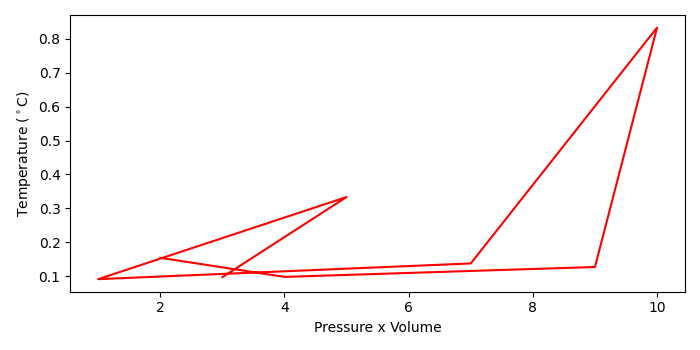# Matplotlib â€“ How to insert a degree symbol into a Python plot?

To insert a degree symbol into a plot, we can use LaTeX representation.

## Steps

• Create data points for pV, nR and T using numpy.
• Plot pV and T using plot() method.
• Set xlabel for pV using xlabel() method.
• Set the label for temperature with degree symbol using ylabel() method.
• To display the figure, use show() method.

## Example

import numpy as np
from matplotlib import pyplot as plt
plt.rcParams["figure.figsize"] = [7.00, 3.50]
plt.rcParams["figure.autolayout"] = True
pV = np.array([3, 5, 1, 7, 10, 9, 4, 2])
nR = np.array([31, 15, 11, 51, 12, 71, 41, 13])
T = np.divide(pV, nR)
plt.plot(pV, T, c="red")
plt.xlabel("Pressure x Volume")
plt.ylabel("Temperature ($^\circ$C)")
plt.show()

## Output